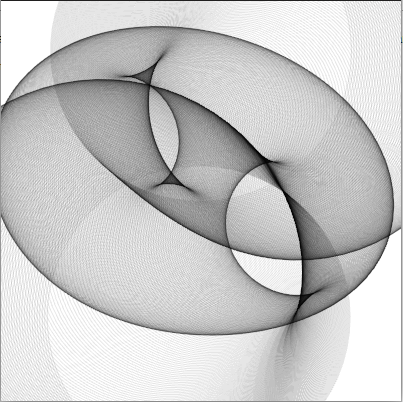## sketch experiment 8 - circles animated

for this sketch experiment I animated the circles i create in experiment 3``````(make-instance 'blub )
(defsketch blub ((title "circles anim") (p 0))
(background (rgb 1 1 1 ))
(incf p 0.1)
(translate 200 50)
(dotimes (i 720)
(with-pen (make-pen :stroke (rgb 0 0 0 0.1 ))
(push-matrix)

(setf f (+ p  (* (sin (* 6.28 (/ i 720)) ) (+ 0 (* 100 (cos (/ i 72.3)))))))
(circle (* 100 (sin (* 3.14 (/ i 180)))) (/ i 2.7) f)
(pop-matrix )
)
)
)
``````